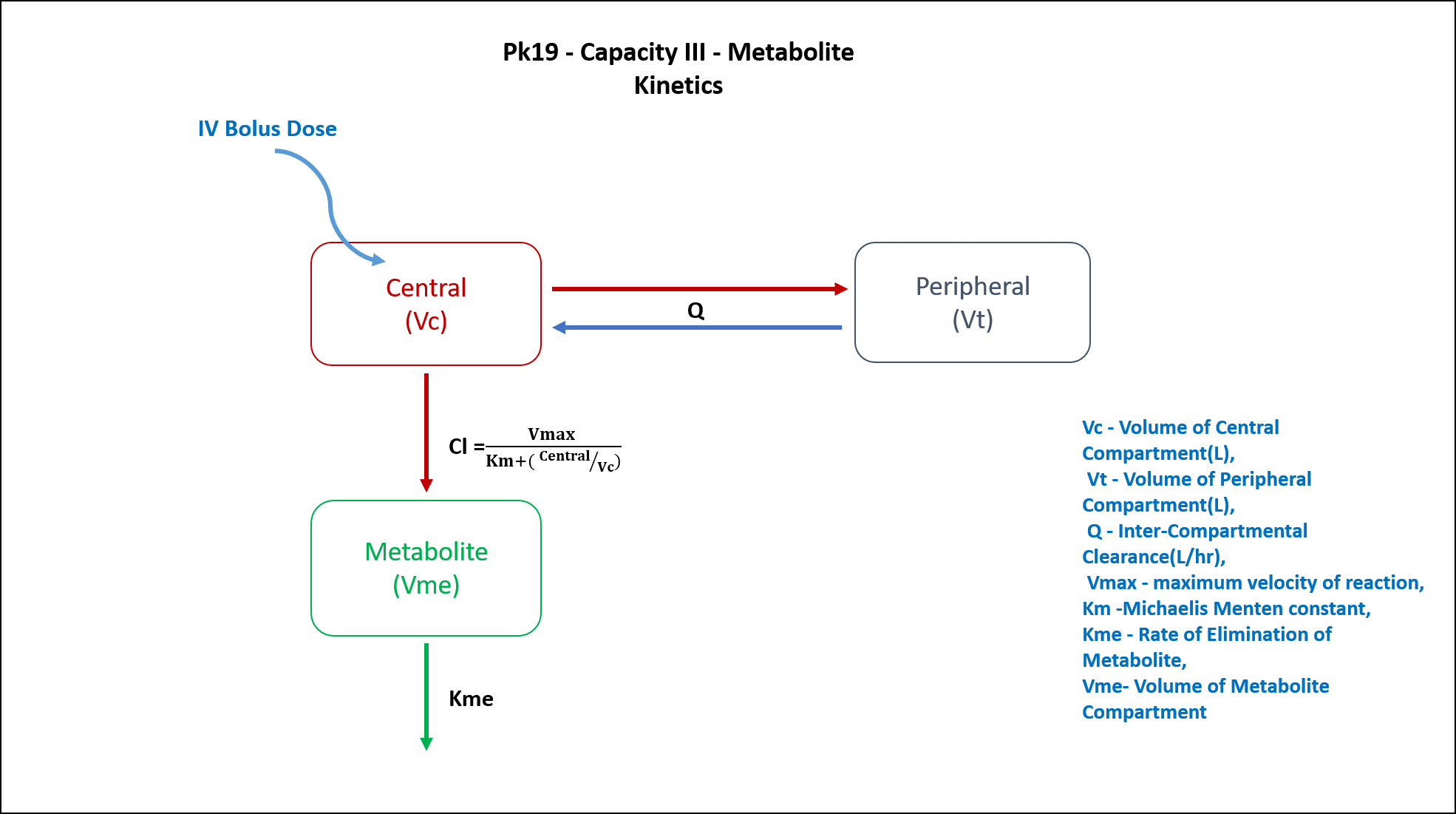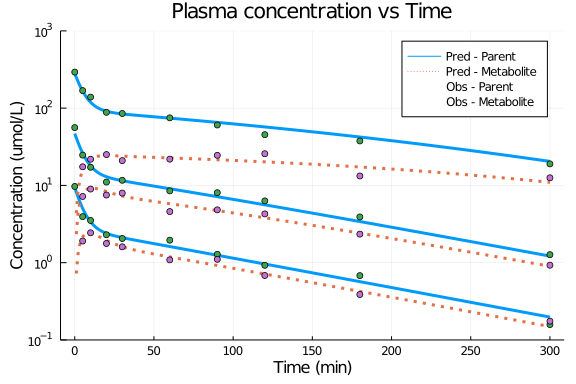# Exercise PK19 - Capacity III - Metabolite Kinetics

### Background

• Structural model - Two Compartment model with a Metabolite Compartment

• Route of administration - IV Bolus

• Dosage Regimen - 10μmol/kg, 50μmol/kg, 300μmol/kg

• Number of Subjects - 3### Learning Outcome

In this model, 3 different dose of the drug given as an IV Bolus to 3 different subjects, will help you estimate metabolite formation rate and elimination rate.

### Objectives

In this tutorial, you will learn how to build two compartment model, a drug undergoing capacity limited metabolite kinetics.

### Libraries

call the "necessary" libraries to get start.

using Pumas
using Plots
using CSV
using StatsPlots
using Random


### Model

In this two compartment model, we administer 3 different doses in 3 different subjects of a drug that undergoes metabolite kinetics.

pk_19           = @model begin
@param begin
tvvc        ∈ RealDomain(lower=0)
tvvp        ∈ RealDomain(lower=0)
tvq         ∈ RealDomain(lower=0)
tvvmax      ∈ RealDomain(lower=0)
tvkm        ∈ RealDomain(lower=0)
tvkme       ∈ RealDomain(lower=0)
tvvme       ∈ RealDomain(lower=0)
Ω           ∈ PDiagDomain(7)
σ²_prop_cp  ∈ RealDomain(lower=0)
σ²_prop_met ∈ RealDomain(lower=0)
end

@random begin
η           ~ MvNormal(Ω)
end

@pre begin
Vc          = tvvc * exp(η)
Vp          = tvvp * exp(η)
Q           = tvq * exp(η)
Vmax        = tvvmax * exp(η)
Km          = tvkm * exp(η)
Kme         = tvkme * exp(η)
Vme         = tvvme * exp(η)
end

@vars begin
VMKM       := Vmax/(Km+(Central/Vc))
end

@dynamics begin
Central'    = -VMKM*(Central/Vc) - (Q/Vc)*Central + (Q/Vp)*Peripheral
Peripheral' = (Q/Vc)*Central - (Q/Vp)*Peripheral
Metabolite' = VMKM*(Central/Vc) - Kme*Metabolite
end

@derived begin
cp          = @. Central/Vc
dv_cp       ~ @. Normal(cp, sqrt(cp^2*σ²_prop_cp))
met         = @. Metabolite/Vme
dv_met      ~ @. Normal(met, sqrt(met^2*σ²_prop_met))
end
end

PumasModel
Parameters: tvvc, tvvp, tvq, tvvmax, tvkm, tvkme, tvvme, Ω, σ²_prop_cp, σ
²_prop_met
Random effects: η
Covariates:
Dynamical variables: Central, Peripheral, Metabolite
Derived: cp, dv_cp, met, dv_met
Observed: cp, dv_cp, met, dv_met


### Parameters

The parameters are as given below. tv represents the typical value for parameters.

• Vc - Volume of Central Compartment (L/kg)

• Vp - Volume of Peripheral Compartment (L/kg)

• Q - Inter-Compartmental Clearance (L/min)

• Vmax - Maximum Velocity of Reaction (μmol/min/kg)

• Km - Michaelis-Menten constant (μmol/L)

• Kme - Rate of Elimination of Metabolite (min⁻¹)

• Vme - Volume of Metabolite Compartment (L/kg)

• Ω - Between Subject Variability

• σ - Residual error

param = ( tvvc        = 1.06405,
tvvp        = 2.00748,
tvq         = 0.128792,
tvvmax      = 1.64429,
tvkm        = 54.794,
tvkme       = 0.145159,
tvvme       = 0.290811,
Ω           = Diagonal([0.0,0.0,0.0,0.0,0.0,0.0,0.0]),
σ²_prop_cp  = 0.015,
σ²_prop_met = 0.015)

(tvvc = 1.06405, tvvp = 2.00748, tvq = 0.128792, tvvmax = 1.64429, tvkm = 5
4.794, tvkme = 0.145159, tvvme = 0.290811, Ω = [0.0 0.0 … 0.0 0.0; 0.0 0.0
… 0.0 0.0; … ; 0.0 0.0 … 0.0 0.0; 0.0 0.0 … 0.0 0.0], σ²_prop_cp = 0.015, σ
²_prop_met = 0.015)


### Dosage Regimen

Three Subjects were adminitered with three different doses of 10μmol/kg, 50μmol/kg and 300μmol/kg.

ev1      = DosageRegimen(10, cmt=1, time=0)
sub1     = Subject(id=1, events=ev1)
ev2      = DosageRegimen(50, cmt=1, time=0)
sub2     = Subject(id=2, events=ev2)
ev3      = DosageRegimen(300, cmt=1, time=0)
sub3     = Subject(id=3, events=ev3)
pop3_sub = [sub1,sub2,sub3]

Population
Subjects: 3
Covariates:


### Simulation

We will simulate the parent plasma concentration and metabolite plasma concentration.

Random.seed!(123)
sim_pop3_sub = simobs(pk_19, pop3_sub, param, obstimes=0:1:300)
df1          = DataFrame(sim_pop3_sub)


### Dataframe & Plot

Use the datafreme for plotting

df1_dv = filter(x -> x.time in [0,5,10,20,30,60,90,120,180,300], df1)

@df df1 plot(:time,:cp, yaxis=:log,
xlabel="Time (min)", ylabel="Concentration (umol/L)" , label="Pred - Parent",
title = "Plasma concentration vs Time", linewidth=3,
ylims=(0.1,1000), yticks=[0.1,1,10,100,1000], xticks=[0,50,100,150,200,250,300])
@df df1 plot!(:time,:met, yaxis=:log,
label="Pred - Metabolite", linestyle=[:dot],linewidth=3)
@df df1_dv scatter!(:time, :dv_cp, yaxis=:log, label="Obs - Parent")
@df df1_dv scatter!(:time, :dv_met, yaxis=:log, label="Obs - Metabolite")